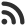Feed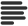Articles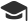Tutorials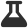Lab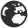CompaniesLeaderboard
 HS Intern at Microsoft Updated Feb. 2, 2020, 12:04 p.m. ⋅ 230 views

# Search an element in a sorted and rotated array

Searching an element in any array is very common task we are asked to do during interviews and in competitive programming contests.

We can search an element in a sorted array by using binary search in O(log n) time complexity. But things become mess when the given array is rotated.

In this article, I'll explain how to search an element in a sorted rotated array efficiently.

The problem here we  are tyring to solve is, we are given an array which is rotated on the basis of any pivot, and we have to find out an element inside it, we are not given the pivot.

### What is sorted rotated array ?

A sorted rotated array is an array whose elements are sorted but, they are rotated with respect to a pivot or key. This key is one of the elements of array. We will not be given the pivot beforehand.

Let's take an example.

``````Sorted array = [1, 2, 3, 4, 5]

rotated array = [3, 4, 5, 1, 2]``````

### How to identify pivot ?

Since, the array is sorted, all the elements are supposed to be in ascending order but pivot is the element will be the one after which next will be smaller.

### Solution

We'll find the pivot and then on the basis of pivot we'll divide the entire array in 2 parts, and since both of the parts will be sorted, we'll call binary search to find elements for each one.

Here is how our method to find pivot would look like:

``````// Function to get pivot.
// returns the position of pivot

int findPivot(int arr[], int low, int high)
{
// base cases
if (high < low) return -1;
if (high == low) return low;

int mid = (low + high)/2; /*low + (high - low)/2;*/
if (mid < high && arr[mid] > arr[mid + 1])
return mid;

if (mid > low && arr[mid] < arr[mid - 1])
return (mid-1);

if (arr[low] >= arr[mid])
return findPivot(arr, low, mid-1);

return findPivot(arr, mid + 1, high);
} ``````

The normal binary search would look like this:

``````// Normal Binary Search

int binarySearch(int arr[], int low,
int high, int key)
{
if (high < low)
return -1;

int mid = (low + high)/2; /*low + (high - low)/2;*/
if (key == arr[mid])
return mid;

if (key > arr[mid])
return binarySearch(arr, (mid + 1), high, key);

return binarySearch(arr, low, (mid -1), key);
} ``````

and this how our final fucntion would look like:

``````int SearchInRotated(int arr[], int n, int key)
{
int pivot = findPivot(arr, 0, n-1);

// If we didn't find a pivot,
// then array is not rotated at all

if (pivot == -1)
return binarySearch(arr, 0, n-1, key);

// If we found a pivot, then first compare with pivot
// and then search in two subarrays around pivot

if (arr[pivot] == key)
return pivot;

if (arr <= key)
return binarySearch(arr, 0, pivot-1, key);

return binarySearch(arr, pivot+1, n-1, key);
} ``````

Let's implement this in a complete program:

``````#include <bits/stdc++.h>
using namespace std;

// Normal Binary Search

int binarySearch(int arr[], int low,
int high, int key)
{
if (high < low)
return -1;

int mid = (low + high)/2; /*low + (high - low)/2;*/
if (key == arr[mid])
return mid;

if (key > arr[mid])
return binarySearch(arr, (mid + 1), high, key);

return binarySearch(arr, low, (mid -1), key);
}

// Function to get pivot.
// returns the position of pivot

int findPivot(int arr[], int low, int high)
{
// base cases
if (high < low) return -1;
if (high == low) return low;

int mid = (low + high)/2; /*low + (high - low)/2;*/
if (mid < high && arr[mid] > arr[mid + 1])
return mid;

if (mid > low && arr[mid] < arr[mid - 1])
return (mid-1);

if (arr[low] >= arr[mid])
return findPivot(arr, low, mid-1);

return findPivot(arr, mid + 1, high);
}

int SearchInRotated(int arr[], int n, int key)
{
int pivot = findPivot(arr, 0, n-1);

// If we didn't find a pivot,
// then array is not rotated at all

if (pivot == -1)
return binarySearch(arr, 0, n-1, key);

// If we found a pivot, then first compare with pivot
// and then search in two subarrays around pivot

if (arr[pivot] == key)
return pivot;

if (arr <= key)
return binarySearch(arr, 0, pivot-1, key);

return binarySearch(arr, pivot+1, n-1, key);
}

int main()
{

int n, key;
int arr;

cout<<"Enter the number of elements in array"<<endl;
cin>>n;

cout<<"Enter n elements for array"<<endl;

for( int i = 0; i < n; i++ )
{

cin>>arr[i];

}

cout<<"What is element to search"<<endl;
cin>>key;

// Call the SeachInRotated

cout << "Index of the element is : " <<
SearchInRotated(arr, n, key);

return 0;
}
``````
``````Enter the number of elements in array
5
Enter n elements for array
3
4
5
1
2
What is element to search
1
Index of the element is : 3 ``````

### Handling duplicates

If we have duplicates in the array then there is no way we can correctly identify the pivot and hence, this apporach will not work, we will have to do normal linear search.

Events

Nov. 28, 2018, 5:30 p.m.

Python from zero to hero

place Delhi

Aug. 13, 2018, 5:30 p.m.

Python from zero to hero

place Bangalore ( HackersFriend office BTM Layout)